This book is archived and will be removed July 6, 2022. Please use the updated version.

Long-Term Liabilities

# 80 Prepare Journal Entries to Reflect the Life Cycle of Bonds

Recall from the discussion in Explain the Pricing of Long-Term Liabilities that one way businesses can generate long-term financing is by borrowing from lenders.

In this section, we will explore the journal entries related to bonds. Earlier, we found that cash flows related to a bond include the following:

1. The receipt of cash when the bond is issued
2. Payment of interest each period
3. Repayment of the bond at maturity

A journal entry must be made for each of these transactions. As we go through the journal entries, it is important to understand that we are analyzing the accounting transactions from the perspective of the issuer of the bond. These are considered long-term liabilities. The investor would make the opposite journal entries. For example, on the issue date of a bond, the borrower receives cash while the lender pays cash.

A final point to consider relates to accounting for the interest costs on the bond. Recall that the bond indenture specifies how much interest the borrower will pay with each periodic payment based on the stated rate of interest. The periodic interest payments to the buyer (investor) will be the same over the course of the bond. It may help to think of personal loan examples. For example, if you or your family have ever borrowed money from a bank for a car or home, the payments are typically the same each month. The interest payments will be the same because of the rate stipulated in the bond indenture, regardless of what the market rate does. The amount of interest cost that we will recognize in the journal entries, however, will change over the course of the bond term, assuming that we are using the effective interest.

Defining Long-Term Liabilities

Under both IFRS and US GAAP, the general definition of a long-term liability is similar. However, there are many types of long-term liabilities, and various types have specific measurement and reporting criteria that may differ between the two sets of accounting standards. With two exceptions, bonds payable are primarily the same under the two sets of standards.

The first difference pertains to the method of interest amortization. Beyond FASB’s preferred method of interest amortization discussed here, there is another method, the straight-line method. This method is permitted under US GAAP if the results produced by its use would not be materially different than if the effective-interest method were used. IFRS does not permit straight-line amortization and only allows the effective-interest method.

The second difference pertains to how the bonds are reported on the books. Under US GAAP, bonds are recorded at face value and the premium or discount is recorded in a separate account. IFRS does not use “premium” or “discount” accounts. Instead, under IFRS, the carrying value of bonds issued at either a premium or discount is shown on the balance sheet at its net. For example, \$100,000 bonds issued at a discount of \$4,000 would be recorded under US GAAP as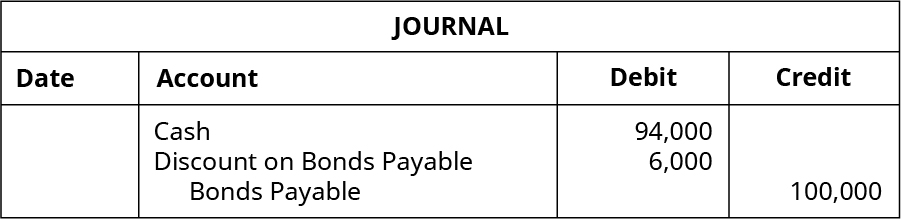Under IFRS, these bonds would be reported as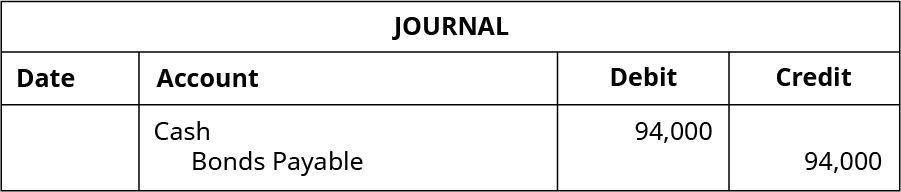Obviously, the above example implies that, in the subsequent entries to recognize interest expense, under IFRS, the Bonds Payable account is amortized directly for the increase or reduction in bond principal. Suppose in this example that the cash interest was \$200 and the interest expense for the first interest period was \$250. The entry to record the transaction under the two different standards would be as follows:

Under US GAAP: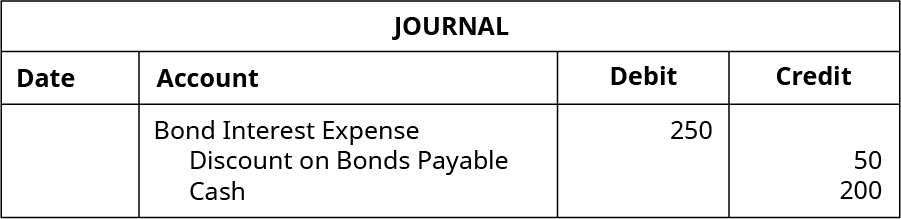Under IFRS: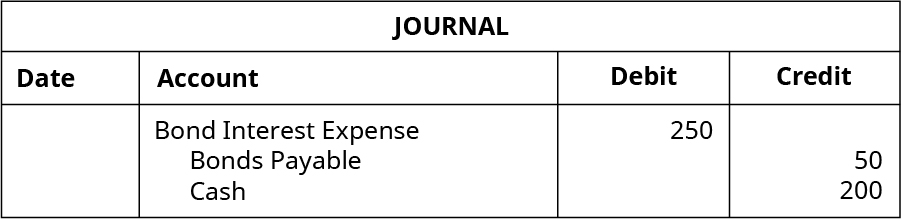Note that under either method, the interest expense and the carrying value of the bonds stays the same.

### Issuance of Bonds

Since the process of underwriting a bond issuance is lengthy and extensive, there can be several months between the determination of the specific characteristics of a bond issue and the actual issuance of the bond. Before the bonds can be issued, the underwriters perform many time-consuming tasks, including setting the bond interest rate. The bond interest rate is influenced by specific factors relating to the company, such as existing debt balances and the ability of the company to repay the funds, as well as the market rate, which is influenced by many external economic factors.

Because of the time lag caused by underwriting, it is not unusual for the market rate of the bond to be different from the stated interest rate. The difference in the stated rate and the market rate determine the accounting treatment of the transactions involving bonds. When the bond is issued at par, the accounting treatment is simplest. It becomes more complicated when the stated rate and the market rate differ.

#### Issued When Market Rate Equals Contract Rate

First, we will explore the case when the stated interest rate is equal to the market interest rate when the bonds are issued.

Returning to our example of a \$1,000, 5-year bond with a stated interest rate of 5%, at issuance, the market rate was 5% and the sales price was quoted at 100, which means the seller of the bond will receive (and the investor will pay) 100% of the \$1,000 face value of the bond. The journal entry to record the sale of 100 of these bonds is: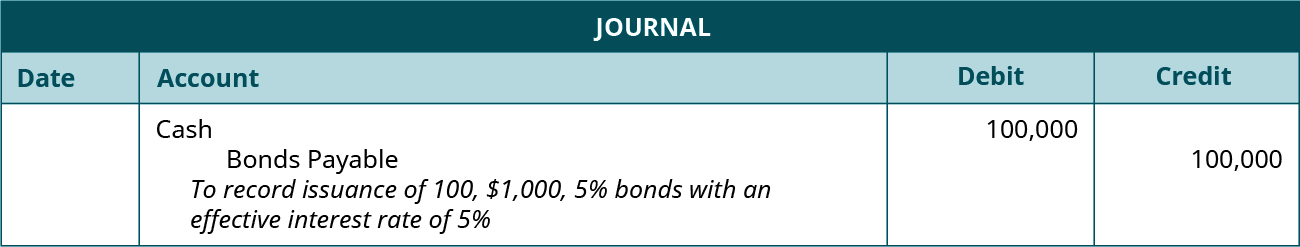Since the book value is equal to the amount that will be owed in the future, no other account is included in the journal entry.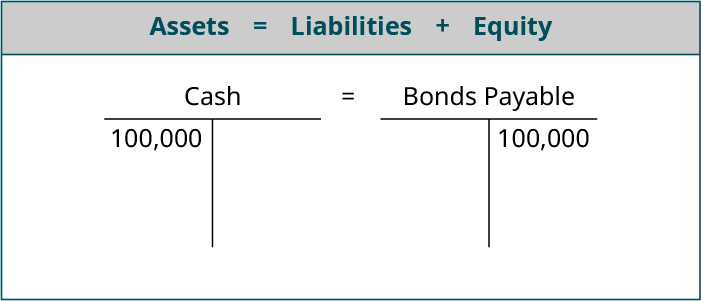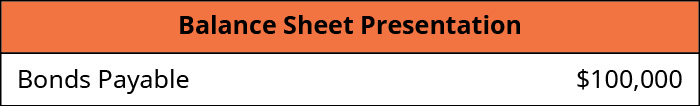If, during the timeframe of establishing the bond stated rate and issuing the bonds, the market rate drops below the stated interest, the bonds would become more valuable. In other words, the investors will earn a higher rate on these bonds than if the investors purchased similar bonds elsewhere in the market. Naturally, investors would want to purchase these bonds and earn a higher interest rate. The increased demand drives up the bond price to a point where investors earn the same interest as similar bonds. Earlier, we found that the sale price of a \$1,000, 5-year bond with a stated rate of 5% and a market rate of 4% is 104.46. That is, the bond will sell at 104.46% of the \$1,000 face value, which means the seller of the bond will receive (and the investor will pay) \$1,044.60.

Selling 100 of these bonds, would yield \$104,460.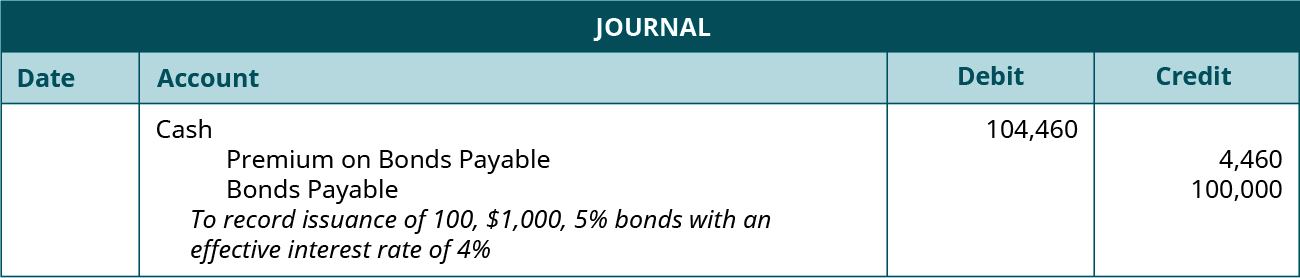The financial statement presentation looks like this: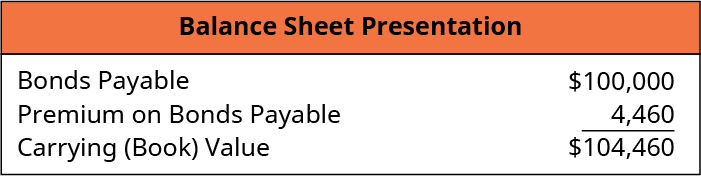On the date that the bonds were issued, the company received cash of \$104,460.00 but agreed to pay \$100,000.00 in the future for 100 bonds with a \$1,000 face value. The difference in the amount received and the amount owed is called the premium. Since they promised to pay 5% while similar bonds earn 4%, the company received more cash up front. In other words, they sold the bond at a premium. They did this because the cost of the premium plus the 5% interest on the face value is mathematically the same as receiving the face value but paying 4% interest. The interest rate was effectively the same.

The premium on bonds payable account is a contra liability account. It is contra because it increases the amount of the Bonds Payable liability account. It is “married” to the Bonds Payable account on the balance sheet. If one of the accounts appears, both must appear. The Premium will disappear over time as it is amortized, but it will decrease the interest expense, which we will see in subsequent journal entries.

Taken together, the Bond Payable liability of \$100,000 and the Premium on Bond Payable contra liability of \$4,460 show the bond’s carrying value or book value—the value that assets or liabilities are recorded at in the company’s financial statements.

The effect on the accounting equation looks like this: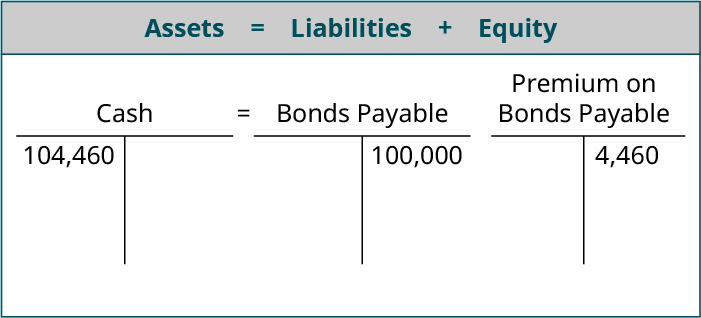It looks like the issuer will have to pay back \$104,460, but this is not quite true. If the bonds were to be paid off today, the full \$104,460 would have to be paid back. But as time passes, the Premium account is amortized until it is zero. The bondholders have bonds that say the issuer will pay them \$100,000, so that is all that is owed at maturity. The premium will disappear over time and will reduce the amount of interest incurred.

#### Issued at a Discount

Bonds issued at a discount are the exact opposite in concept as bonds issued at a premium. If, during the timeframe of establishing the bond stated rate and issuing the bonds, the market rate rises above the stated interest on the bonds, the bonds become less valuable because investors can earn a higher rate of interest on other similar bonds. In other words, the investors will earn a lower rate on these bonds than if the investors purchased similar bonds elsewhere in the market. Naturally, investors would not want to purchase these bonds and earn a lower interest rate than could be earned elsewhere. The decreased demand drives down the bond price to a point where investors earn the same interest for similar bonds. Earlier, we found the sale price of a \$1,000, 5-year bond with a stated interest rate of 5% and a market rate of 7% is 91.80. That is, the bond will sell at 91.80% of the \$1,000 face value, which means the seller of the bond will receive (and the investor will pay) \$918.00. On selling 100 of the \$1,000 bonds today, the journal entry would be: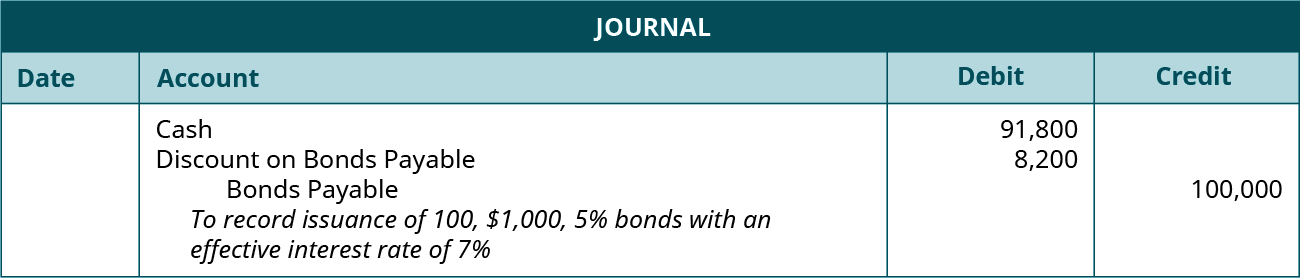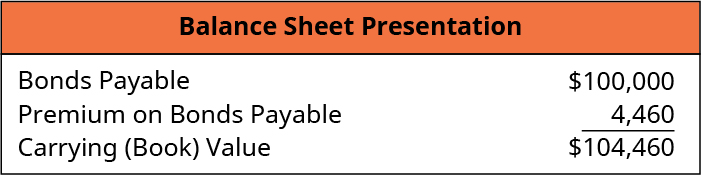Today, the company receives cash of \$91,800.00, and it agrees to pay \$100,000.00 in the future for 100 bonds with a \$1,000 face value. The difference in the amount received and the amount owed is called the discount. Since they promised to pay 5% while similar bonds earn 7%, the company, accepted less cash up front. In other words, they sold the bond at a discount. They did this because giving a discount but still paying only 5% interest on the face value is mathematically the same as receiving the face value but paying 7% interest. The interest rate was effectively the same.

Like the Premium on Bonds Payable account, the discount on bonds payable account is a contra liability account and is “married” to the Bonds Payable account on the balance sheet. The Discount will disappear over time as it is amortized, but it will increase the interest expense, which we will see in subsequent journal entries.

The effect on the accounting equation looks like this: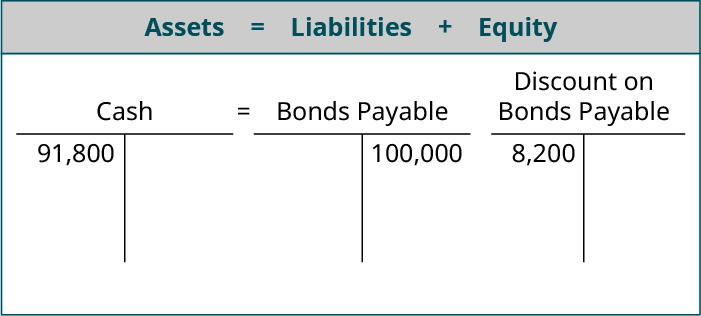### First and Second Semiannual Interest Payment

When a company issues bonds, they make a promise to pay interest annually or sometimes more often. If the interest is paid annually, the journal entry is made on the last day of the bond’s year. If interest was promised semiannually, entries are made twice a year.

Municipal Bonds

Municipal bonds are a specific type of bonds that are issued by governmental entities such as towns and school districts. These bonds are issued in order to finance specific projects (such as water treatment plants and school building construction) that require a large investment of cash. The primary benefit to the issuing entity (i.e., the town or school district) is that cash can be obtained more quickly than, for example, collecting taxes and fees over a long period of time. This allows the project to be completed sooner, which is a benefit to the community.

Municipal bonds, like other bonds, pay periodic interest based on the stated interest rate and the face value at the end of the bond term. However, corporate bonds often pay a higher rate of interest than municipal bonds. Despite the lower interest rate, one benefit of municipal bonds relates to the tax treatment of the periodic interest payments for investors. With corporate bonds, the periodic interest payments are considered taxable income to the investor. For example, if an investor receives \$1,000 of interest and is in the 25% tax bracket, the investor will have to pay \$250 of taxes on the interest, leaving the investor with an after-tax payment of \$750. With municipal bonds, interest payments are exempt from federal tax. So the same investor receiving \$1,000 of interest from a municipal bond would pay no income tax on the interest income. This tax-exempt status of municipal bonds allows the entity to attract investors and fund projects more easily.

#### Interest Payment: Issued When Market Rate Equals Contract Rate

Recall that the Balance Sheet presentation of the bond when the market rate equals the stated rate is as follows:In this example, the company issued 100 bonds with a face value of \$1,000, a 5-year term, and a stated interest rate of 5% when the market rate was 5% and received \$100,000. As previously discussed, since the bonds were sold when the market rate equals the stated rate, the carrying value of the bonds is \$100,000. These bonds did not specify when interest was paid, so we can assume that it is an annual payment. If the bonds were issued on January 1, the company would pay interest on December 31 and the journal entry would be: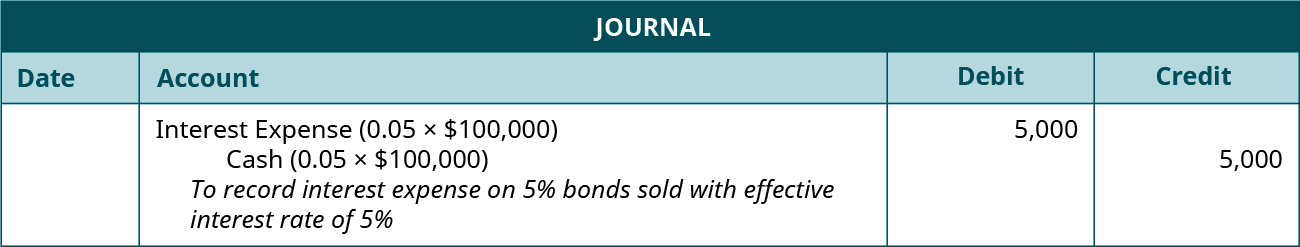The interest expense is calculated by taking the Carrying Value (\$100,000) multiplied by the market interest rate (5%). The stated rate is used when calculating the interest cash payment. The company is obligated by the bond indenture to pay 5% per year based on the face value of the bond. When the situation changes and the bond is sold at a discount or premium, it is easy to get confused and incorrectly use the market rate here. Since the market rate and the stated rate are the same in this example, we do not have to worry about any differences between the amount of interest expense and the cash paid to bondholders. This journal entry will be made every year for the 5-year life of the bond.

When performing these calculations, the rate is adjusted for more frequent interest payments. If the company had issued 5% bonds that paid interest semiannually, interest payments would be made twice a year, but each interest payment would only be half an annual interest payment. Earning interest for a full year at 5% annually is the equivalent of receiving half of that amount each six months. So, for semiannual payments, we would divide 5% by 2 and pay 2.5% every six months.

Mortgage Debt

According to Statista the amount of mortgage debt—debt incurred to purchase homes—in the United States was \$14.9 trillion on 2017. This value does not include the interest cost—the cost of borrowing—related to the debt.

A common loan term for those borrowing money to buy a house is 30 years. Each month, the borrower must make payments on the loan, which would add up to 360 payments for a 30-year loan. Recall from previous discussions on amortization that each payment can be divided into two components: the interest expense and the amount that is applied to reduce the principal.

In order to calculate the amount of interest and principal reduction for each payment, banks and borrowers often use amortization tables. While amortization tables are easily created in Microsoft Excel or other spreadsheet applications, there are many websites that have easy-to-use amortization tables. The popular lending website Zillow has a loan calculator to calculate the monthly payments of a loan as well as an amortization table that shows how much interest and principal reduction is applied for each payment.

For example, borrowing \$200,000 for 30 years at an interest rate of 5% would require the borrow to repay a total \$386,513. The monthly payment on this loan is \$1,073.64. This amount represents the \$200,000 borrowed and \$186,513 of interest cost. If the borrower chose a 15-year loan, the total payments drops significantly to \$266,757, but the monthly payments increase to \$1,581.59.

Because interest is calculated based on the outstanding loan balance, the amount of interest paid in the first payment is much more than the amount of interest in the final payment. The pie charts below show the amount of the \$1,073.64 payment allocated to interest and loan reduction for the first and final payments, respectively, on the 30-year loan.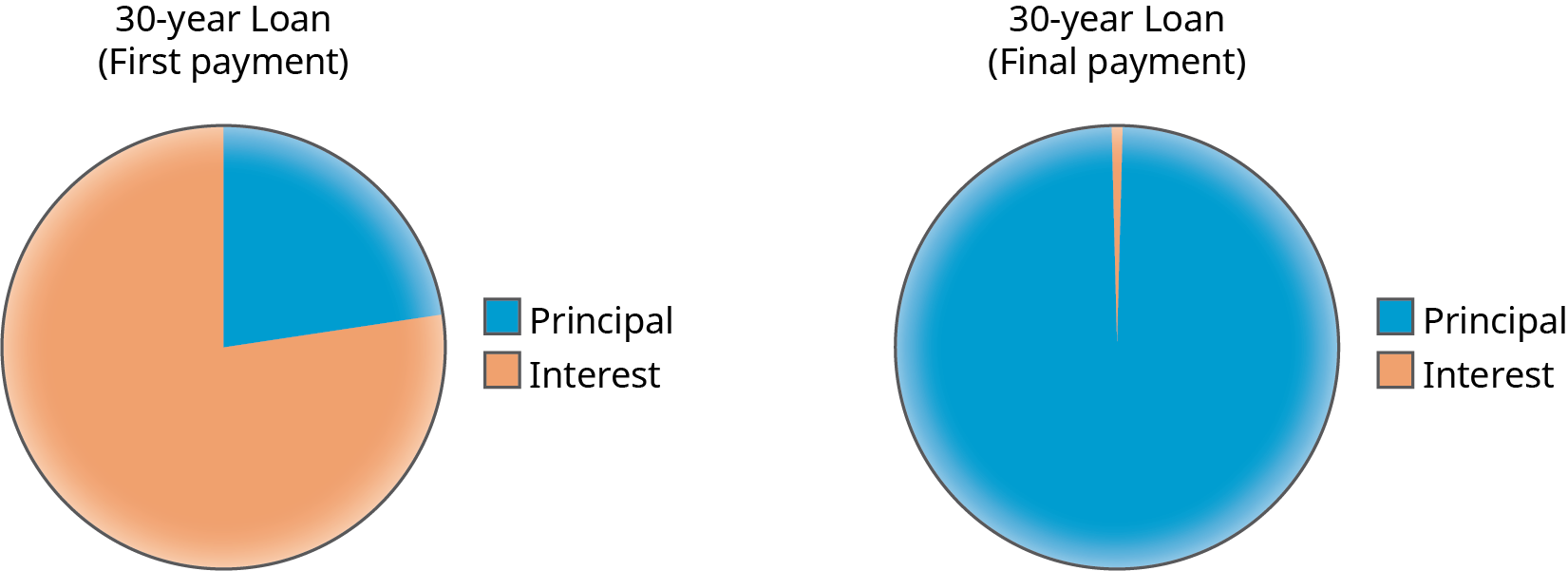#### Interest Payment: Issued at a Premium

Recall that the Balance Sheet presentation of the bond when the market rate at issue is lower than the stated rate is as follows:In this scenario, the sale price of a \$1,000, 5-year bond with a stated rate of 5% and a market rate of 4% was \$1,044.60. If the company sold 100 of these bonds, it would receive \$104,460 and the journal entry would be: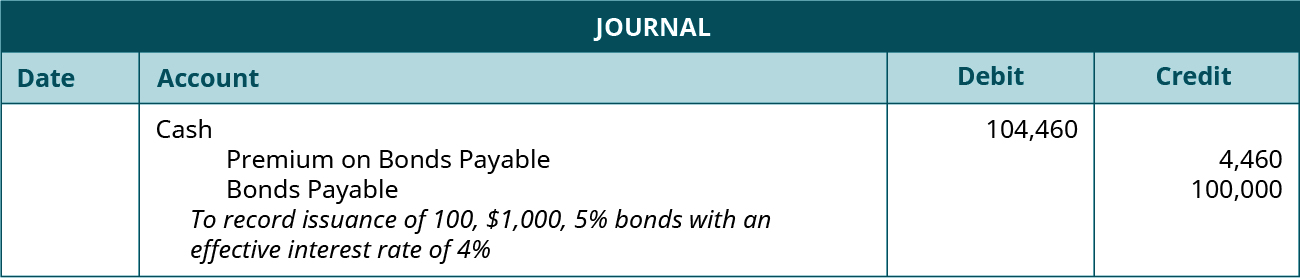Again, let’s assume that the bonds pay interest annually. At the end of the bond’s year, we would record the interest expense: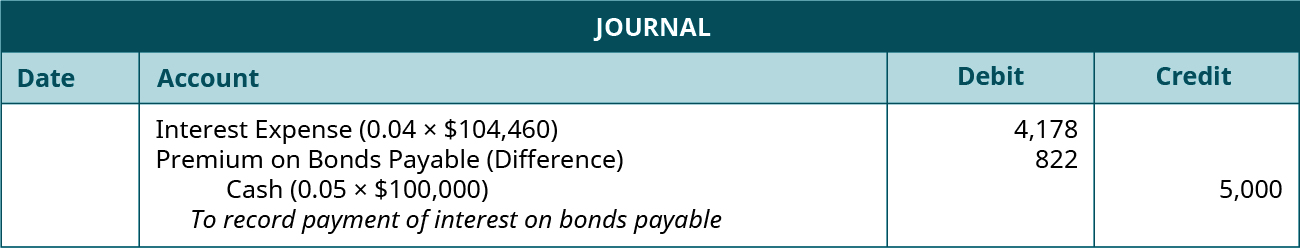The interest expense determination is calculated using the effective interest amortization interest method. Under the effective-interest method, the interest expense is calculated by taking the Carrying (or Book) Value (\$104,460) multiplied by the market interest rate (4%). The amount of the cash payment in this example is calculated by taking the face value of the bond (\$100,000) multiplied by the stated rate.

Since the market rate and the stated rate are different, we need to account for the difference between the amount of interest expense and the cash paid to bondholders. The amount of the premium amortization is simply the difference between the interest expense and the cash payment. Another way to think about amortization is to understand that, with each cash payment, we need to reduce the amount carried on the books in the Bond Premium account. Since we originally credited Bond Premium when the bonds were issued, we need to debit the account each time the interest is paid to bondholders because the carrying value of the bond has changed. Note that the company received more for the bonds than face value, but it is only paying interest on \$100,000.

The partial effect of the first period’s interest payment on the company’s accounting equation in year one is: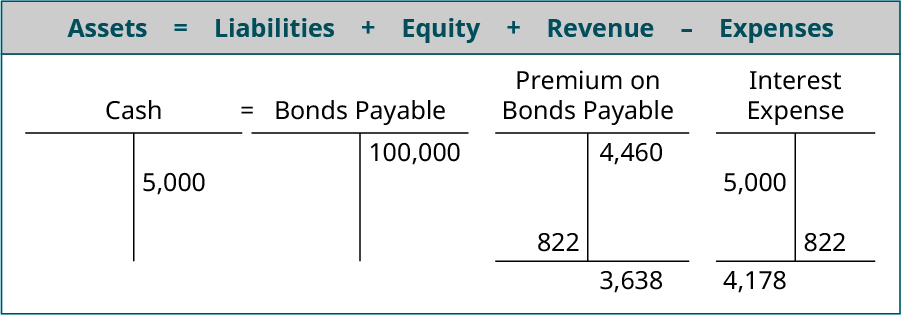And the financial-statement presentation at the end of year 1 is: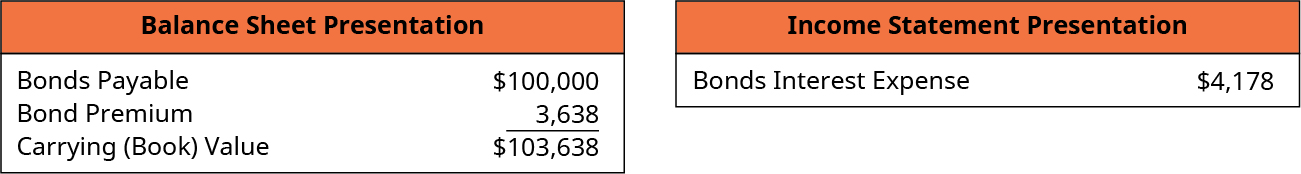The journal entry for year 2 is: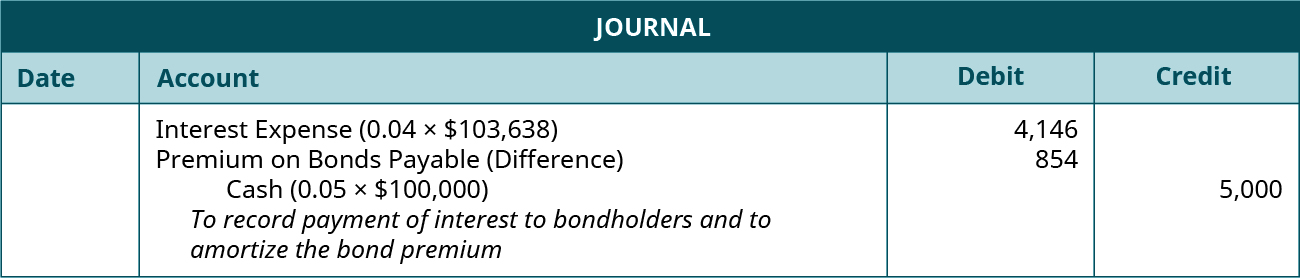The interest expense is calculated by taking the Carrying (or Book) Value (\$103,638) multiplied by the market interest rate (4%). The amount of the cash payment in this example is calculated by taking the face value of the bond (\$100,000) multiplied by the stated rate (5%). Since the market rate and the stated rate are different, we again need to account for the difference between the amount of interest expense and the cash paid to bondholders.

The partial effect on the accounting equation in year two is: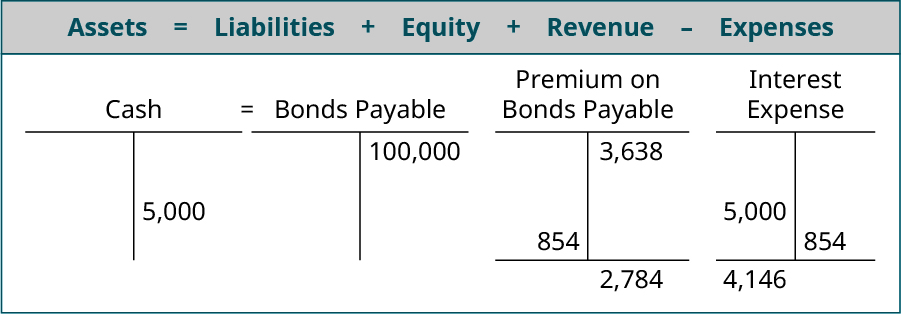And the financial-statement presentation at the end of year 2 is: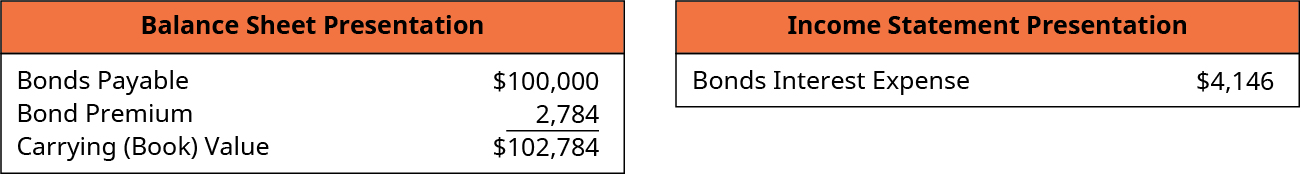By the end of the 5th year, the bond premium will be zero, and the company will only owe the Bonds Payable amount of \$100,000.

#### Interest Payment: Issued at a Discount

Recall that the Balance Sheet presentation of the bond when the market rate at issue was higher than the stated rate is as follows: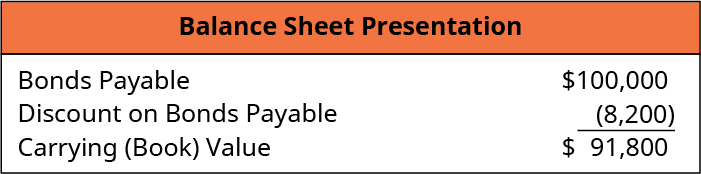We found the sale price of a \$1,000, 5-year bond with a stated interest rate of 5% and a market rate of 7% was \$918.00. We then showed the journal entry to record sale of 100 bonds: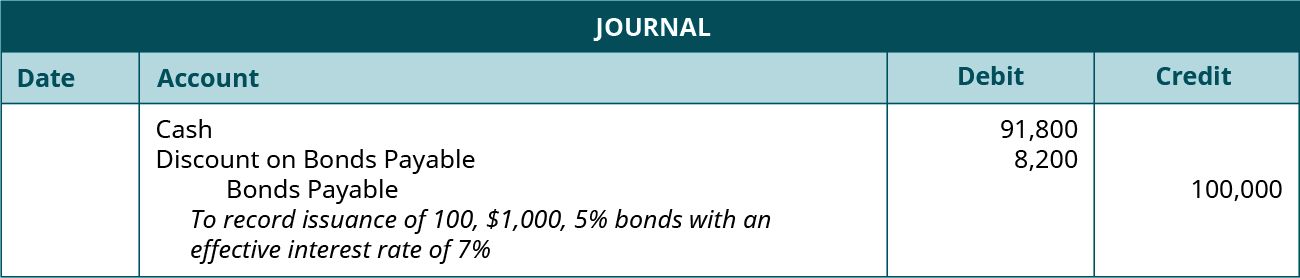At the end of the bond’s first year, we make this journal entry: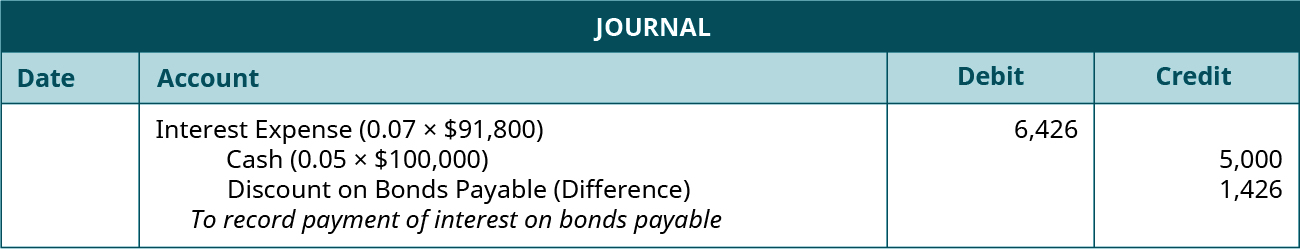The interest expense is calculated by taking the Carrying Value (\$91,800) multiplied by the market interest rate (7%). The amount of the cash payment in this example is calculated by taking the face value of the bond (\$100,000) and multiplying it by the stated rate (5%). Since the market rate and the stated rate are different, we need to account for the difference between the amount of interest expense and the cash paid to bondholders. The amount of the discount amortization is simply the difference between the interest expense and the cash payment. Since we originally debited Bond Discount when the bonds were issued, we need to credit the account each time the interest is paid to bondholders because the carrying value of the bond has changed. Note that the company received less for the bonds than face value but is paying interest on the \$100,000.

The partial effect on the accounting equation in year one is: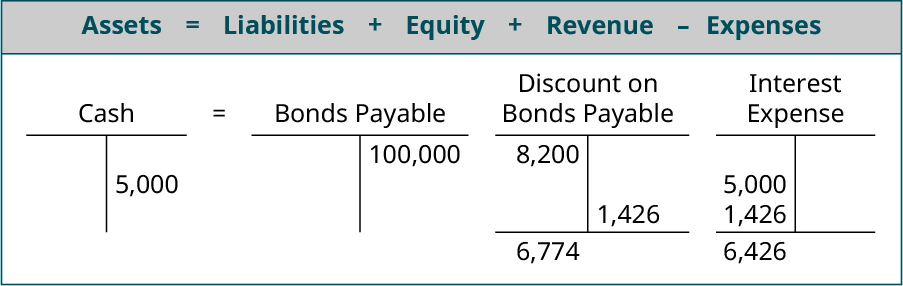And the financial-statement presentation at the end of year 1 is: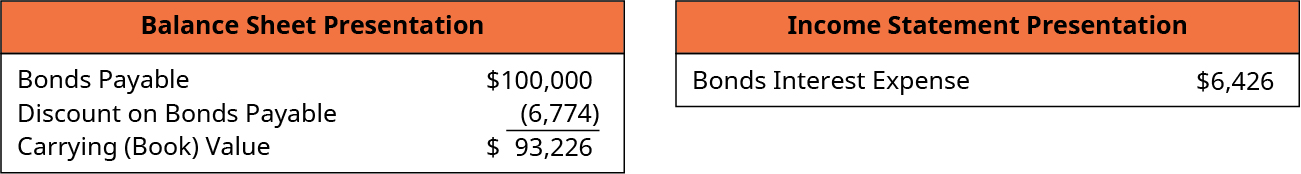The journal entry for year 2 is: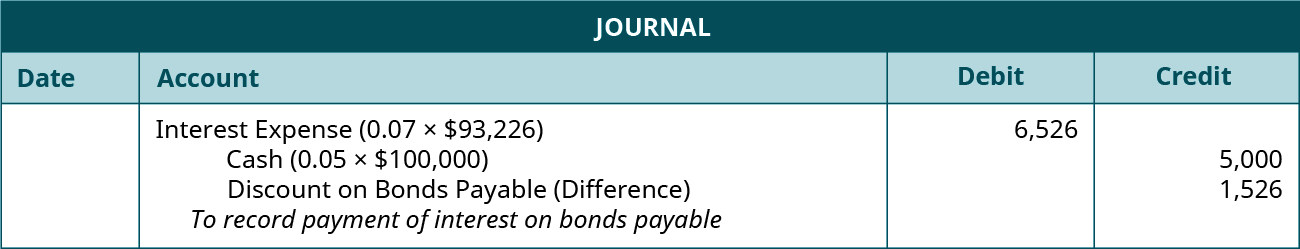The interest expense is calculated by taking the Carrying Value (\$93,226) multiplied by the market interest rate (7%). The amount of the cash payment in this example is calculated by taking the face value of the bond (\$100,000) multiplied by the stated rate (5%). Again, we need to account for the difference between the amount of interest expense and the cash paid to bondholders by crediting the Bond Discount account.

The partial effect on the accounting equation in year two is: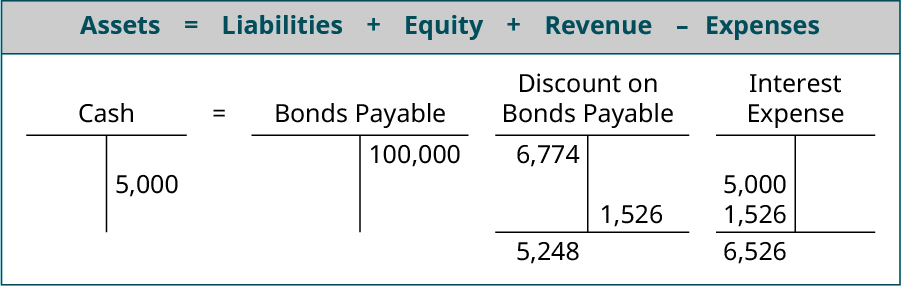And the financial statement presentation at the end of year 2 is: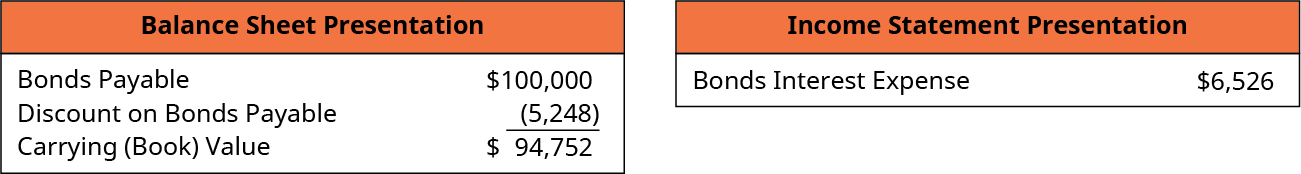By the end of the 5th year, the bond premium will be zero and the company will only owe the Bonds Payable amount of \$100,000.

### Retirement of Bonds When the Bonds Were Issued at Par

At some point, a company will need to record bond retirement, when the company pays the obligation. Often, they will retire bonds when they mature. For example, earlier we demonstrated the issuance of a five-year bond, along with its first two interest payments. If we had carried out recording all five interest payments, the next step would have been the maturity and retirement of the bond. At this stage, the bond issuer would pay the maturity value of the bond to the owner of the bond, whether that is the original owner or a secondary investor.

This example demonstrates the least complicated method of a bond issuance and retirement at maturity. There are other possibilities that can be much more complicated and beyond the scope of this course. For example, a bond might be callable by the issuing company, in which the company may pay a call premium paid to the current owner of the bond. Also, a bond might be called while there is still a premium or discount on the bond, and that can complicate the retirement process. Situations like these will be addressed in later accounting courses.

To continue with our example, assume that the company issued 100 bonds with a face value of \$1,000, a 5-year term, and a stated interest rate of 5% when the market rate was 5% and received \$100,000. It was recorded in this way:At the end of 5 years, the company will retire the bonds by paying the amount owed. To record this action, the company would debit Bonds Payable and credit Cash. Remember that the bond payable retirement debit entry will always be the face amount of the bonds since, when the bond matures, any discount or premium will have been completely amortized.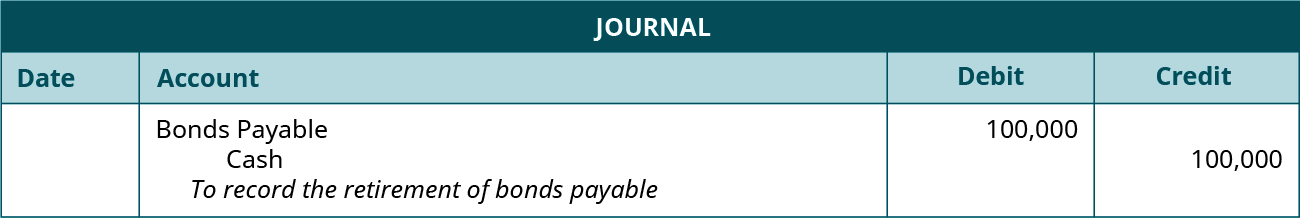### Key Concepts and Summary

• When a company issues a bond, the specific terms of the bond are contained in the bond indenture.
• Journal entries are recorded at various stages of a bond, including when the bond is issued, for periodic interest payments, and for payment of the bond at maturity.
• The difference between the face value of a bond and the cash proceeds are recorded in the discount (when the proceeds are lower than the face value) and premium (when the proceeds are higher than the face value) accounts.
• The carrying or book value of a bond is determined by the balances of the Bond Payable and Discount and/or Premium accounts.
• Interest expense associated with a bond interest payment is calculated by the bond’s carrying or book value multiplied by the market interest rate.

### Multiple Choice

(Figure)Naval Inc. issued \$200,000 face value bonds at a discount and received \$190,000. At the end of 2018, the balance in the Discount on Bonds Payable account is \$5,000. This year’s balance sheet will show a net liability of ________.

1. \$200,000
2. \$180,000
3. \$195,000
4. \$205,000

C

(Figure)Keys Inc. issued 100 bonds with a face value of \$1,000 and a rate of 8% at \$1,025 each. The journal entry to record this transaction includes ________.

1. a credit to Bonds Payable for \$102,500
2. a credit to cash for \$102,500
3. a debit to cash for \$100,000
4. a credit to Premium on Bonds Payable for \$2,500

(Figure)Huang Inc. issued 100 bonds with a face value of \$1,000 and a 5-year term at \$960 each. The journal entry to record this transaction includes ________.

1. a debit to Bonds Payable for \$100,000
2. a debit to Discount on Bonds Payable for \$4,000
3. a credit to cash for \$96,000
4. a credit to Discount on Bonds Payable for \$4,000

B

(Figure)O’Shea Inc. issued bonds at a face value of \$100,000, a rate of 6%, and a 5-year term for \$98,000. From this information, we know that the market rate of interest was ________.

1. more than 6%
2. less than 6%
3. equal to 6%
4. cannot be determined from the information given.

(Figure)Gingko Inc. issued bonds with a face value of \$100,000, a rate of 7%, and a 10-yearterm for \$103,000. From this information, we know that the market rate of interest was ________.

1. more than 7%
2. less than 7%
3. equal to 7%
4. equal to 1.3%

B

### Questions

(Figure)If there is neither a premium nor discount present, the journal entry to record bond interest payments is _______.

DR Interest Expense and CR Cash

(Figure)When do you use the Bond Discount Account?

(Figure)A company issued bonds with a \$100,000 face value, a 5-year term, a stated rate of 6%, and a market rate of 7%. Interest is paid annually. What is the amount of interest the bondholders will receive at the end of the year?

Bondholders receive the stated rate times the principle, so they would receive \$6,000.

(Figure)A company issued \$100,000, 5-year bonds, receiving \$97,000. What is the balance sheet presentation immediately after the sale?

(Figure)Does interest expense increase or decrease when a bond premium is amortized?

Amortizing a bond premium reduces interest expense.

### Exercise Set A

(Figure)On Jan. 1, Year 1, Foxcroft Inc. issued 100 bonds with a face value of \$1,000 for \$104,000. The bonds had a stated rate of 6% and paid interest semiannually. What is the journal entry to record the issuance of the bonds?

(Figure)Medhurst Corporation issued \$90,000 in bonds for \$87,000. The bonds had a stated rate of 8% and pay interest quarterly. What is the journal entry to record the sale of the bonds?

(Figure)On Jan. 1, Year 1, Foxcroft Inc. issued 100 bonds with a face value of \$1,000 for \$104,000. The bonds had a stated rate of 6% and paid interest semi-annually. What is the journal entry to record the first payment to the bondholders?

(Figure)Pinetop Corporation issued \$150,000 10-year bonds at par. The bonds have a stated rate of 6% and pay interest annually. What is the journal entry to record the sale of the bonds?

(Figure)Medhurst Corporation issued \$90,000 in bonds for \$87,000. The bonds had a stated rate of 8% and pay interest quarterly. What is the journal entry to record the first interest payment?

### Exercise Set B

(Figure)Willoughby Inc. issued 100 bonds with a face value of \$1,000 and a stated rate of 4% and received \$105,000. What is the journal entry to record the sale of the bonds?

(Figure)Allante Corporate issued 50 bonds with a face value of \$1,000 and a stated rate of 4% and received \$45,000. What is the journal entry to record the sale of the bonds?

(Figure)Roo Incorporated issued 50 bonds with a face value of \$1,000 and a stated rate of 6% when the market rate was 6%. What is the journal entry to record the sale of the bonds?

(Figure)Piedmont Corporation issued \$200,000 of 10-year bonds at par. The bonds have a stated rate of 6% and pay interest annually. What is the journal entry to record the first interest payment to the bondholders?

(Figure)Lunar Corporation issued \$80,000 in bonds for \$87,000 on Jan. 1. The bonds had a stated rate of 8% and pay interest quarterly. What is the journal entry to record the first interest payment?

### Problem Set A

(Figure)Volunteer Inc. issued bonds with a \$500,000 face value, 10% interest rate, and a 4-year term on July 1, 2018 and received \$540,000. Interest is payable annually. The premium is amortized using the straight-line method. Prepare journal entries for the following transactions.

1. July 1, 2018: entry to record issuing the bonds
2. June 30, 2019: entry to record payment of interest to bondholders
3. June 30, 2019: entry to record amortization of premium
4. June 30, 2020: entry to record payment of interest to bondholders
5. June 30, 2020: entry to record amortization of premium

(Figure)Aggies Inc. issued bonds with a \$500,000 face value, 10% interest rate, and a 4-year term on July 1, 2018, and received \$540,000. Interest is payable semi-annually. The premium is amortized using the straight-line method. Prepare journal entries for the following transactions.

1. July 1, 2018: entry to record issuing the bonds
2. Dec. 31, 2018: entry to record payment of interest to bondholders
3. Dec. 31, 2018: entry to record amortization of premium

### Problem Set B

(Figure)Dixon Inc. issued bonds with a \$500,000 face value, 10% interest rate, and a 4-year term on July 1, 2018 and received \$480,000. Interest is payable annually. The discount is amortized using the straight-line method. Prepare journal entries for the following transactions.

1. July 1, 2018: entry to record issuing the bonds
2. June 30, 2019: entry to record payment of interest to bondholders
3. June 30, 2019: entry to record amortization of discount
4. June 30, 2020: entry to record payment of interest to bondholders
5. June 30, 2020: entry to record amortization of discount

(Figure)Edward Inc. issued bonds with a \$500,000 face value, 10% interest rate, and a 4-year term on July 1, 2018 and received \$480,000. Interest is payable semiannually. The discount is amortized using the straight-line method. Prepare journal entries for the following transactions.

1. July 1, 2018: entry to record issuing the bonds
2. Dec. 31, 2018: entry to record payment of interest to bondholders
3. Dec. 31, 2018: entry to record amortization of discount

### Thought Provokers

(Figure)Assume you are a newly hired accountant for a local manufacturing firm. You have enjoyed working for the company and are looking forward to your first experience participating in the preparation of the company’s financial statements for the year-ending December 31, the end of the company’s fiscal year.

As you are preparing your assigned journal entries, your supervisor approaches you and asks to speak with you. Your supervisor is concerned because, based on her preliminary estimates, the company will fall just shy of its financial targets for the year. If the estimates are true, this means that all 176 employees of the company will not receive year-end bonuses, which represent a significant portion of their pay.

One of the entries that you will prepare involves the upcoming bond interest payment that will be paid on January 15 of the next year. Your supervisor has calculated that, if the journal entry is dated on January 1 of the following year rather than on December 31 of the current year, the company will likely meet its financial goals thereby allowing all employees to receive year-end bonuses. Your supervisor asks you if you will consider dating the journal entry on January 1 instead of December 31 of the current year. Assess the implications of the various stakeholders and explain what your answer will be.

### Glossary

book value
difference between the asset’s value (cost) and accumulated depreciation; also, value at which assets or liabilities are recorded in a company’s financial statements
discount on bonds payable
contra liability account associated with a bond that has a stated rate that is lower than the market rate and is sold at a discount